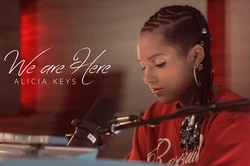## FANDOM

42 Pages"We Are Here" is a single from Alicia Keys's sixth album.

## LyricsEdit

We are here
We are here for all of us
We are here for all of us
That's why we are here, why we are here
We are here

So real but it's so sad
And while we burnin' this incense, we gon' pray for the innocent
Cause right now it don't make sense
Right now it don't make sense
Cause right now it is real
Let's talk about, let's talk Nigeria
In a mass hysteria, yeah
Our souls are brought together so that we could love each other
Brother,

We are here
We are here for all of us
We are here for all of us
That's why we are here, why we are here
We are here

No guns made in Harlem, but yet crime is a problem
He wanna shine, they wanna rob him
Single mother, where they come from?
How we gonna save the nation, with no support for education
Cause right now it don't make sense
Right now it don't make sense
Let's do more giving
Do more forgiving, yeah
Our souls were brought together so that we could love each other
Sister,

We are here
We are here for all of us
We are here for all of us
That's why we are here, why we are here
We are here
We are here for all of us
We are here for all of us
That's why we are here, why we are here
We are here
Oh oh oh, oh oh oh oh
Oh oh oh, oh oh oh oh
Oh, oh

Oh oh oh, oh oh oh oh
Oh oh oh oh oh oh oh
Oh, oh

We are here (oh oh oh, oh oh oh oh)
We are here for all of us (oh oh oh, oh oh oh oh)
(oh, oh)
We are here for all of us (oh oh oh, oh oh oh oh)
(oh oh oh, oh oh oh oh)
that's why we are here, why we are here (oh, oh)

Cause we are here
We are here for all of us
We are here for all of us
That's why we are here, why we are here
We are, here
We are here for all of us
We are here for all of us
That's why we are here,
Why we are here